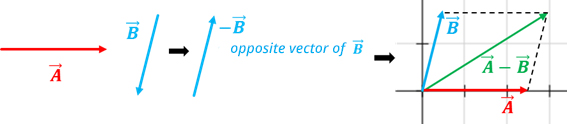# addition and subtraction of vectors

Html code here! Replace this with any non empty text and that's it.The  sum y subtraction two vector A  y B, results in another vector, that is to say,

A + B = C   y   AB = C

For the addition and subtraction of vectors different methods are applied depending on whether they have the same direction or not. The main methods are: the direct method, the triangle method and the parallelogram.

## Vector sum

To add two vectors A  y B adds A  with vector BThat is, the components of each vector are added:

A + B = (Ax + Bx, TOy + By, TOz + Bz)

Example: Sean A = (3, 2, -4) and B = (-3, 2, 7), calculate the vector A + B.

A + B = ( 3 + (-3), 2 + 2, -4 – 7) = (0, 4, 3)

### Sum of two vectors with the same direction and the same direction

1. We draw the vector B below the vector A, so that they are consecutive, respecting their modules, directions and directions.
2. The vector adds A + B it has as a module the sum of the modules of both, the same direction and the same direction of the given vectors.The resulting vector A + B has the sum of A  and of B, the same direction and the same sense as A  y B.

### Sum of two vectors with the same direction and the opposite direction

1. We draw the vector B below the vector A, so that it is consecutive, respecting its modules, directions and directions.
2. The vector sum has as a module the difference of the modules of both, the same direction and the direction of the larger vector.The resulting vector A + B has as a module the difference of A  and of B, the same direction and the same sense as A  y B.

### Sum of two vectors with different directions

To add two vectors A  y B forming an angle to each other, two methods are used: the triangle method and the parallelogram method.

#### Triangle method

1. We draw the vectors consecutively, that is, the origin of B has to match the end A.
2. The vector adds A + B has as its origin the origin of A  and as an extreme, that of B.#### Parallelogram law or method

1. We draw the vector A at the origin of a Cartesian plane respecting its module, direction and direction.
2. We draw at the origin of A, the vector B respecting its module, direction and sense.
3. Lines are drawn parallel to each vector forming a parallelogram.
4. The resulting vector will be the diagonal of the parallelogram starting at the origin of the Cartesian plane.## Vector subtraction

To subtract two vectors A y B adds A with the opposite of vector B, that is to say:

A - B = A + (- B)

The components of the vector A - B are obtained by subtracting its components.

A - B = (Ax - Bx, TOy - By, TOz - Bz)

Example: Be A = (5, 2, 4) and B = (-3, 5, 9), calculate the vector A - B.

A - B = ( 5-(-3), 2-5, 4-9) = (8,-3,-5)

### Opposite vector method

To subtract two vectors A y B:

1. Like vector B is the subtraction we must draw its opposite vector; therefore we draw a vector equal to B but in the opposite direction.
2. We apply the parallelogram law.

#### Triangle method

1. We draw at the origin of A, the vector B respecting its module, direction and sense.
2. The resulting vector A - B will originate from the end of B (vector subtracting) and as extreme, the extreme of A  (minuend vector).